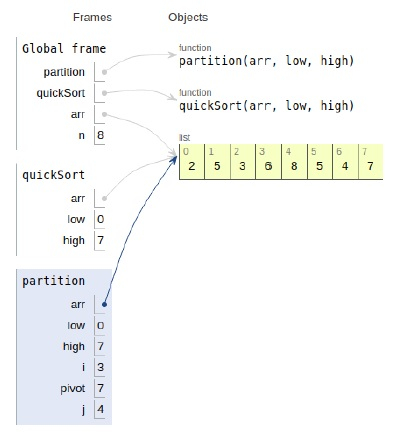# Python Program for QuickSort

PythonServer Side ProgrammingProgramming

Problem statement − We are given an array, we need to sort it using the concept of quicksort

Here we first partition the array and sort the separate partition to get the sorted array.

Now let’s observe the solution in the implementation below −

## Example

Live Demo

# divide function
def partition(arr,low,high):
i = ( low-1 )
pivot = arr[high] # pivot element
for j in range(low , high):
# If current element is smaller
if arr[j] <= pivot:
# increment
i = i+1
arr[i],arr[j] = arr[j],arr[i]
arr[i+1],arr[high] = arr[high],arr[i+1]
return ( i+1 )
# sort
def quickSort(arr,low,high):
if low < high:
# index
pi = partition(arr,low,high)
# sort the partitions
quickSort(arr, low, pi-1)
quickSort(arr, pi+1, high)
# main
arr = [2,5,3,8,6,5,4,7]
n = len(arr)
quickSort(arr,0,n-1)
print ("Sorted array is:")
for i in range(n):
print (arr[i],end=" ")

## Output

Sorted array is
2 3 4 5 5 6 7 8All the variables are declared in the local scope and their references are seen in the figure above.